# MP Board Class 8th Maths Solutions Chapter 3 Understanding Quadrilaterals Ex 3.3

## MP Board Class 8th Maths Solutions Chapter 3 Understanding Quadrilaterals Ex 3.3

Question 1.
Given a parallelogram ABCD. Complete each statement along with the definition or property used.(i) AD = …..
(ii) ∠DCB = …….
(iii) OC = ……..
(iv) m∠DAB + m∠CDA =
Solution:
Given that ABCD is a parallelogram.
(i) AD = BC [∵ In a parallelogram opposite sides are equal]
(ii) ∠DCB = ∠DAB [∵ In a parallelogram opposite angles are equal]
(iii) OC = OA [∵ In a parallelogram diagonals bisect each other]
(iv) m∠DAB + m∠CDA = 180° [∵ Adjacent angles in a parallelogram are supplementary]Question 2.
Consider the following parallelograms. Find the values of the unknowns x, y, z.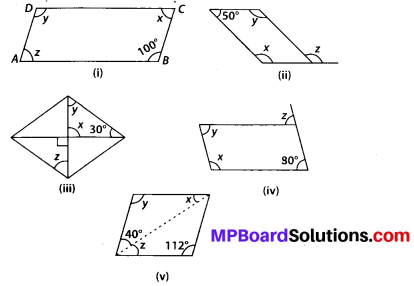Solution:
(i) ABCD is a parallelogram in which ∠B = 100° (given)
∴ ∠A + ∠B = 180° [∵ Sum of adjacent angles is 180°]
⇒ z +100° = 180°
⇒ z = 180° – 100° = 80°
Also ∠B = ∠D and ∠A = ∠C
[∵ Opposite angles are equal]
∴ ∠B = 100° = ∠D = y and ∠A = z = 80° = ∠C = x
∠ x = 80°, y = 100°, z = 80°.

(ii) y + 50° = 180° [∵ Sum of adjacent angles is 180°]
⇒ y = 180° – 50° = 130°
Also, y = x = 130° [∵ Opposite angles are equal in a parallelogram]
And 180°- z = 50° [Linear pair]
⇒ z = 180°- 50° ⇒ z = 130°

(iii) Clearly, x = 90°
[∵ Vertical opposite angles are equal] Also, x + y + 30° = 180°
[By using angle sum property of a triangle]
⇒ 90° + 30° + y = 180°
⇒ 120° + y = 180° ⇒ y = 180° – 120° = 60°
Since, alternate angles are equal in a parallelogram
∴ y = z = 60°
Thus x = 90°, y = 60° and z = 60°.

(iv) Since x + 80° = 180°
[Sum of adjacent angles is 180°] ⇒ x = 180° – 80° = 100°
Also 80° = y [∵ Opposite angles are equal in a parallelogram]
And x = 180° – z ⇒ 100° = 180° – 2° ⇒ 2 = 180° – 100° = 80°
Thus x = 100°, y = 80° and 2 = 80°.

(v) y = 112° [∵ Opposite angles are equal in a parallelogram]
y + x + 40° = 180°
[By angle sum property of a triangle]
⇒ 112°+ x+ 40° = 180°
⇒ x +152° = 180° ⇒ x = 180° – 152°
⇒ x = 28°
⇒ x = 2 = 28° [∵ Alternate angles are equal in a parallelogram]Question 3.
Can a quadrilateral ABCD be a parallelogram if
(i) ∠D + ∠B = 180°?
(ii) AB = DC = 8 cm, AD = 4 cm and BC = 4.4 cm?
(iii) ∠A = 70° and ∠C = 65°?
Solution:
(i) Yes, but need not be true.
(ii) No, because in a parallelogram opposite sides are equal but here AD ≠ BC.
(iii) No, because in a parallelogram opposite angles are equal but here ∠A ≠ ∠C.

Question 4.
Draw a rough figure of a quadrilateral that is not a parallelogram but has exactly two opposite angles of equal measure.
Solution:We can draw a figure of a kite in which exactly two opposite angles are equal.
Hence ∠D = ∠B.Question 5.
The measures of two adjacent angles of a parallelogram are in the ratio 3 : 2. Find the measure of each of the angles of the parallelogram.
Solution:
Let ABCD be a given parallelogram.
Let ∠A = 3x and ∠B = 2x.
Since the sum of adjacent angles in a parallelogram is 180°
∴ m∠A + m∠B = 180°
⇒ 3x + 2x = 180°
⇒ 5x = 180° ⇒ x = 36°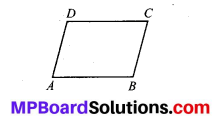∴ m∠A = 3 × 36° = 108° and m∠B = 2 × 36° = 72°
Also, m∠A = m∠C = 108°
[∵ In a parallelogram opposite angles are equal]
and m∠B = m∠D = 72°.Question 6.
Two adjacent angles of a parallelogram have equal measure. Find the measure of each of the angles of the parallelogram.
Solution:
Let ABCD be a parallelogram, in which, let m∠A = m∠B = x
Since sum of two adjacent angles is 180°
∴ m∠A + m∠B = 180°
⇒ x + x = 180° ⇒ 2x = 180°
⇒ x = 90°
Also m∠A = m∠C = 90°
[∵ In a parallelogram opposite angles are equal]
and m∠B = m∠D = 90°.

Question 7.
The given figure HOPE is a parallelogram. Find the angle measures x, y and z. State the
properties you use to find them.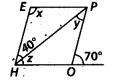Solution:
y = 40°
[Since PO || HE ∴ alternate angles are equal]
70° = y + 2 [Exterior angle property of a triangle]
⇒ z = 70° – 40° = 30°
∠POH = 180° – 70° = 110° [Linear pair]
∠POH = x = 110° [Opposite angles are equal]
Thus x = 110°, y = 40°, 2 = 30°.Question 8.
The following figures GUNS and RUNS are parallelograms. Find x and y. (Lengths are in cm)Solution:
(i) Since GUNS is a parallelogram.
∴ GS = NU and GU = SN
[∵ In a parallelogram opposite sides are equal]
⇒ 3x = 18 ⇒ x = 6
and 3y -1 = 26 ⇒ 3y = 1 + 26 = 27
⇒ y = 9
Thus x = 6 cm and y = 9 cm.

(ii) Since diagonals bisects each other in a parallelogram.
So, 20 = y + 7 ⇒ y = 13
Also, x + y= 16 ⇒ x = 16 – 13 = 3
Thus x = 3 cm and y = 13 cm.

Question 9.
In the given figure both RISK and CLUE are parallelograms. Find the value of x.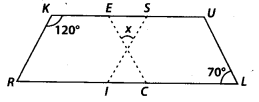Solution:
Since RISK and CLUE are parallelograms.
∴ ∠SKR = ∠RIS = 120° [∵ Opposite angles are equal]
Also, ∠ULC = ∠UEC = 70° [Opposite angles are equal]
∠RIS + ∠ISK = 180° [Adjacent angles are supplementary]
⇒ ∠ISK = 180° – 120° = 60°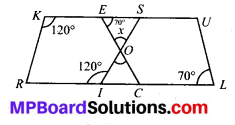In ∆OES, we have
70° + x + 60° = 180° [Angle sum property]
⇒ x + 130° = 180°
⇒ x = 180° – 130° = 50°.Question 10.
Explain how this figure is a trapezium. Which of its two sides are parallel?Solution:
In a trapezium, only one pair of opposite sides are parallel, whereas other pair of opposite sides are non-parallel.
∴ KLMN is a trapezium because MN || KL.
[∵ Sum of two adjacent interior angles is 180° = ( 80° + 100°)].

Question 11.
Find m∠C in given figure if AB || DC.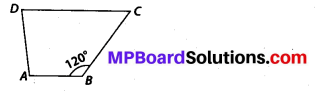Solution:
We are given, $$\overline{A B} \| \overline{D C}$$
∴ Sum of two adjacent interior angles is 180°
i. e., ∠B + ∠C = 180° ⇒ 120° + ∠C= 180°
⇒ ∠C = 180° -120° = 60°.
Thus ∠C = 60°.Question 12.
Find the measure of ∠P and ∠S if $$\overline{S P} \| \overline{R Q}$$ in figure. (If you find m∠R, is there more than one method to find m∠P?)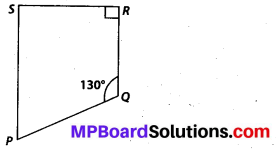Solution:
Since $$\overline{S P} \| \overline{R Q}$$
Thus ∠R + ∠S = 180°
[∵ Sum of two adjacent interior angles is 180°]
⇒ 90° + ∠S = 180° ⇒ ∠S = 180° – 90° = 90°
∠P + ∠Q + ∠R + ∠S = 360° [∵ Sum of all angles of a quadrilateral is 360°]
⇒ ∠P + 310° = 360°
⇒ ∠P = 360° – 310°
⇒ ∠P = 50°
Thus ∠P = 50° and ∠S = 90°.
Also m∠P can be found as ∠P + ∠Q = 180°
[Adjacent angles are supplementary]
⇒ ∠P + 130° = 180°
⇒∠P = 180° -130° ⇒ ∠P = 50°